# Ionogram inversion for MARSIS topside sounding

## Abstract

In the present paper, we propose a method of ionogram inversion to retrieve the electron density profile, Ne(h), of the Martian ionosphere from the topside ionogram, which is measured by the Mars Advanced Radar for Subsurface and Ionosphere Sounding (MARSIS) instrument on board the Mars Express spacecraft. The new inversion technique is developed from Titheridge’s method by replacing the prior polynomials with empirical orthogonal functions (EOFs), which are estimated from the archived Ne(h) observation by the radio occultation of Mars Global Surveyor (MGS). The EOF-based technique has achieved quick convergence and good stability. It is concluded that the newly developed method is an alternative tool for the analysis of MARSIS ionograms.

## 1. Introduction

An ionogram is a representation of data from modern and classical ionosondes as a two-dimensional image, displaying the ionospheric echo intensity versus radio frequency, f, and time delay (or apparent height) of the radio propagation. Through the ionospheric dispersion, the echo apparent height, h′(f), as a function of radio frequency, is determined by the unknown height profile, Ne(h), of the ionospheric electron density. Thus, it is possible to compute Ne(h) from h′(f), which is usually digitized directly from an ionogram. The computation of the electron density profile is usually referred to as an inversion.

Many methods of ionogram inversion have been proposed and developed since the ionosonde was put into use in the 1930s. The initial theory of calculating Ne(h) from h′(f), known as Abel’s integral equation, is given by Whittaker and Watson (1927). Budden (1961) proposed an analytical inversion of Abel’s integral equation which describes the vertical radio wave propagation in an isotropic ionosphere. When the anisotropy is considered, the inversion becomes quite complicated, and lamination methods might be used (Jackson, 1969). However, a lamination method needs a complete h′(f) trace with high frequency resolution, and cannot include the valleys (such as the E-F valley) in Ne(h), whereas some valley model incorporated in the polynomial methods may be used to solve the valley problem. Therefore, the lamination methods are now rarely used in practice. Titheridge (1961, 1967a, b, 1969, 1975, 1988) proposed a model-fitting method, or polynomial analysis in which the curve of true height vs. plasma frequency, h(fp), is represented as a single or overlapping polynomial(s). Huang and Reinisch (1982) further developed the polynomial analysis by replacing Titheridge’s Taylor polynomials with shifted Chebyshev polynomials, and applied it to the data processing of ISIS topside ionograms. Reinisch and Huang (1983) further applied this method in the analysis of ground-based Digisonde ionograms. Recently, researchers have developed the traditional polynomial analysis by replacing the polynomials (Taylor or shifted Chebyshev polynomials) with empirical orthogonal functions (EOFs). For example, Ding et al. (2007) have used the EOF series to represent the electron density profile in the ionospheric F layer at Wuhan, China.

The Mars Advanced Radar for Subsurface and Ionosphere Sounding (MARSIS) aboard the Mars Express is the first topside sounder designed to characterize the interactions of the solar wind with the ionosphere and upper atmosphere of Mars. The details of the MARSIS instrument and the Mars Express spacecraft have been described by Picardi et al. (2004), Chicarro et al. (2004) and Nielsen (2004). Up to now, MARSIS has recorded a large number of topside ionograms, most of which remain to be inverted or well-inverted. The inversion method frequently used, at present, for these MARSIS ionograms is the lamination method (Nielsen et al., 2006; Morgan et al., 2008; Zou et al., 2010). Meanwhile, the analytical inversion of Abel’s integral equation is also used to give the analytical expression of the electron density profiles (Gurnett et al., 2008). In this work, we adopt the EOF series to represent the electron density profile in the Martian topside ionosphere. In the following, Section 2 briefly describes the MARSIS ionograms and their scaling. Section 3 discusses the EOF-based inversion. In Section 4, our inversion technique is applied to the data of six MARSIS ionograms.

## 2. MARSIS Ionograms and Apparent Range Scaling

Figure 1 displays several topside ionograms obtained by MARSIS at different solar zenith angles (SZAs), latitudes and local true solar time (LST). For a detailed description of the MARSIS ionograms, one can refer to Gurnett et al. (2008) and Morgan et al. (2008).

In Figs. 1(a)(f), the strong vertical lines near the left edge of the ionogram represent harmonics of the local plasma frequency and are caused by the excitation of electrostatic oscillations at the local plasma frequency. By measuring the spacing between these harmonics, the local plasma frequency can be determined and will be used later in our inversion. The technique for measuring the local plasma frequency is discussed in detail by Duru et al. (2008).

As shown in Figs. 1(a)(f), in general, the ionospheric echo appears with a time delay that increases with increasing frequency and terminates in a well-defined cusp region. The cusp of the echo trace is caused by the rather long time delay that occurs as the sounding frequency is very close to the peak ionospheric plasma frequency. Hence, the peak plasma frequency of the ionosphere can be determined from the echo cusp.

A semi-automated process, similar to that proposed by Morgan et al. (2008), is used to extract h′(f) from the ionospheric echo of the ionogram. The scaled apparent ranges are indicated by the red crosses, as shown in Figs. 1(a)(f).

## 3. Inversion Procedure

In this paper, we develop an inversion procedure by replacing the polynomials (Taylor or shifted Chebyshev polynomials) with EOFs which are calculated from the archived electron density profiles measured by Mars Global Surveyor (MGS) radio occultation. These archived electron density profiles are available on the website http://nova.stanford.edu/projects/mgs/eds-public.html.

### 3.1 The archived data and the EOF analysis

About 5600 MGS electron density profiles covering high SZAs (70 to 90°), high latitudes (60.5 to 85.7°N and 61.5 to 69.4°S) and LST (2.76 to 14.74 h) are used in the EOF analysis. We first transform these electron density profiles into profiles,, of the normalized plasma frequency,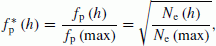(1)

where fp(max) and Ne(max) are the peak values of the plasma frequency, fp, and the corresponding electron density, Ne, respectively, and the superscript asterisk denotes the normalized value.

Assuming that the electron density profile in the Martian topside ionosphere is a monotonically decreasing function, we can obtain a true height functionby inverting the normalized profile. Thus, a sampled dataset ofis obtained for the EOF analysis (Wilks, 1995),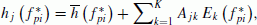(2)

whereis the stepped normalized frequency,is the jth true height function,is the mean height function averaged for all,is the kth EOF (a base function) and A jk is the corresponding coefficient for the jth true height function. The total number of EOFs, K, is determined by the number of.’s are empirically determined by diagonalizing the covariance matrix between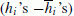; i.e.is the kth orthonormalized eigenvector of the covariance matrix. As shown by standard mathematical analysis (see, e.g., Jolliffe, 2002), if we project the data), if we project the dataonto the’s, then the projection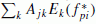, (k = 1, 2, …, K; MK) represents the maximum possible fraction of the variability contained in. Therefore, the EOF series in Eq. (2) converges most quickly in representing the true height dataset. For instance, four leading terms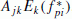, (k = 1, 2, 3, 4) may represent 94% of the total variance of our true height dataset. Hence, in our ionogram inversion we truncated the EOF series at K = 4. The mean height and the four leading EOFs vs. the normalized plasma frequency are illustrated in Fig. 2. The beginning ofis chosen as 0.2 to make sure that all values of the true height are sampled adequately to give a statistically valid result, though the information of the true height at though the information of the true height at smaller than 0.2 will be lost. It is clearly seen that, in general, the mean height function represents the typical variation of the 5600 MGS electron density profiles. The larger rank EOF refers to the smaller scale variation. The different scales of the true height variations can be represented by different ranks of the EOF.

### 3.2 Ionogram inversion

It is assumed that the Martian ionosphere is horizontally stratified. For a vertical incidence radio wave with frequency f, the echo is reflected from the range z r where the plasma frequency fp equals f. In this case, the apparent range from the spacecraft to z r is given by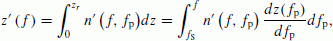(3)

whereis the group refractive index; fS is the local plasma frequency at the spacecraft; z(fp) is the true range, and can be computed from the true height,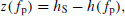(4)

where hS is the true height of the spacecraft. The integral function (Eq. (3)) has a unique solution under the assumption, as was made above, that the electron density distribution in the topside ionosphere of Mars has a monotonic profile.

In the integration of Eq. (3), we adopt the variable transformation, fp = f sin φ, to avoid the infinite n′ at the reflection point. Then Eq. (3) becomes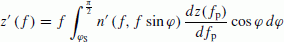(5a)

or equivalently,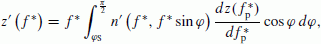(5b)

where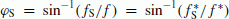. In Eq. (5b),is the normalized plasma frequency defined in Eq. (1). A similar definition is made for the normalized radio frequency that f* = f/fp(max).

Noticing the relationship between the true range and the true height in Eq. (4), the following results may be obtained by substituting Eq. (2) into (5b)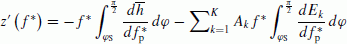(6)

or, in discrete form,(7)

where(8)

can be calculated in advance;is measured from the ionogram with the scaling process in Section 2. Thus, the coefficients A k are estimated by solving the matrix equation (Eq. (7)), and the final electron density profile is then obtained by Eq. (2). However, in most cases, the densities between the spacecraft and the first reflection point are not known. In order to carry out the inversion, we assume that the electron density varies exponentially with height in the gap. The assumption used here, similar to that of Nielsen et al. (2006), Gurnett et al. (2008) and Morgan et al. (2008), is also a good approximation but not a complete description.

In theory, the EOF series, the same as the polynomials used previously (such as the Taylor or shifted Chebyshev polynomials), can be used to expand any electron density profile in the Martian topside ionosphere. Moreover, the EOF series converges more quickly, especially when it is used to represent the electron density profile in the range of the MGS radio occultation data, because the EOF series is derived from the measured MGS radio occultation data.

## 4. Results

The inversion technique was applied to the measured data in Figs. 3(a)(f). In Figs. 3(a)(f) the solid curves show the true height profiles of the normalized plasma frequency. As a check on the overall accuracy of the inversion procedure, we recalculated the apparent height vs. frequency using the calculated profile, and then superimposed the recalculated apparent heights on the measured ones. As can be seen in Figs. 3(a)(f), the agreement of the recalculated apparent heights (circles) with the measured ones (crosses) is very good, indicating that the inversion result is a good estimation of the true electron density distribution because the solution of the inversion problem is unique, as we mentioned in Section 3.

In Fig. 3, the recalculated apparent heights match the measured ones well, whether the electron density profile is in the range of the MGS dataset or not, which indicates that the EOF expansion of the electron density profile, though not optimal, is near optimal when the four EOFs are extrapolated to apply at all points in the topside ionosphere of Mars. The good extrapolation inspires confidence in processing a large quantity of MARSIS ionograms using the method developed.

## 5. Summary and Conclusions

The EOF-based inversion is developed from the traditional polynomial analysis of Titheridge (1961, 1967a, b, 1969, 1975, 1988) and Huang and Reinisch (1982) where the prior polynomials, e.g., Taylor polynomials or shifted Chebyshev polynomials, have now been replaced with empirical orthogonal functions (EOFs). The new technique has been applied here to the data processing of the MARSIS ionograms, with EOFs retrieved from the MGS radio occultation measurements. The results show the remarkable advantage that only a few EOFs are required to represent most of the variability of the original dataset, owing to the quick convergence of the EOF series. These results show that the EOF-based inversion provides a new tool for the analysis of MARSIS ionograms.

## References

• Budden, K. G., Radio Waves in the Ionosphere, Cambridge Univ. Press, Cambridge, U.K., 1961.

• Chicarro, A., P. Martin, and R. Trautner, The Mars Express mission: An overview, in Mars Express: A European Mission to the Red Planet, edited by A. Wilson, pp. 3–16, ESA Publ. Div., Noordwijk, Netherlands, 2004.

• Ding, Z., B. Ning, W. Wan, and L. Liu, Automatic scaling of F2-layer parameters from ionograms based on the empirical orthogonal function (EOF) analysis of ionospheric electron density, Earth Planets Space, 59, 51–58, 2007.

• Duru, F., D. A. Gurnett, D. D. Morgan, R. Modolo, A. F. Nagy, and D. Najib, Electron densities in the upper ionosphere of Mars from the excitation of electron plasma oscillations, J. Geophys. Res., 113, A07302, doi:10.1029/2008JA013073, 2008.

• Gurnett, D. A., R. L. Huff, D. D. Morgan, A. M. Persoon, T. F. Averkamp, D. L. Kirchner, F. Duru, F. Akalin, A. J. Kopf, E. Nielsen, A. Safaeinili, J. J. Plaut, and G. Picardi, An overview of radar soundings of the Martian ionosphere from the Mars Express spacecraft, Adv. Space Res., 41, 1335–1346, doi:10.1016/j.asr.2007.01.062, 2008.

• Huang, X. Q. and B. W. Reinisch, Automatic calculation of electron density profiles from digital ionograms: 2. True height inversion of topside ionograms with the profile-fitting method, Radio Sci., 17, 837–844, 1982.

• Jackson, J. E., The reduction of topside ionograms to electron-density profiles, Proc, IEEE, 57, 960–975, 1969.

• Jolliffe, I. T., Principal Component Analysis, 2nd Ed., Springer, 2002.

• Morgan, D. D., D. A. Gurnett, D. L. Kirchner, J. L. Fox, E. Nielsen, and J. J. Plaut, Variation of the Martian ionospheric electron density from Mars express radar soundings, J. Geophys. Res., 113, A09303, doi:10.1029/2008JA013313, 2008.

• Nielsen, E., Mars Express and MARSIS, Space Sci. Rev., 111, 245–262, 2004.

• Nielsen, E., H. Zou, D. A. Gurnett, D. L. Kirchner, D. D. Morgan, R. Huff, R. Orosei, A. Safaeinili, J. J. Plaut, and G. Picardi, Observations of vertical reflections from the topside Martian ionosphere, Space Sci. Rev., 126, 373–388, doi:10.1007/s11214-006-9113-y, 2006.

• Picardi, G. et al., MARSIS: Mars Advanced Radar for Subsurface and Ionosphere Sounding, in Mars Express: A European Mission to the Red Planet, edited by A. Wilson, pp. 51–70, ESA Publ. Div., Noordwijk, Netherlands, 2004.

• Reinisch, B. W. and X. Q. Huang, Automatic calculation of electron density profiles from digital ionograms: 3. Processing of bottomside ionograms, Radio Sci., 18, 477–492, 1983.

• Titheridge, J. E., A new method for the analysis of ionospheric h′(f) records, J. Atmos. Terr. Phys., 21, 1–12, 1961.

• Titheridge, J. E., The overlapping-polynomial analysis of ionograms, Radio Sci., 2, 1169–1175, 1967a.

• Titheridge, J. E., Direct manual calculation of ionospheric parameters using a single-polynomial analysis, Radio Sci., 2, 1237–1254, 1967b.

• Titheridge, J. E., The single polynomial analysis of ionograms, Radio Sci., 4, 41–51, 1969.

• Titheridge, J. E., The relative accuracy of ionogram analysis techniques, Radio Sci., 10, 589–599, 1975.

• Titheridge, J. E., The real height analysis of ionograms: A generalized formulation, Radio Sci., 23, 831–849, 1988.

• Whittaker, E. T. and G. N. Watson, A Course of Modern Analysis, Cambridge Univ. Press, Cambridge, U.K., 1927.

• Wilks, D. S., Statistical Methods in the Atmospheric Sciences, Academic Press, San Diego, 1995.

• Zou, H., E. Nielsen, J.-S. Wang, and X.-D Wang, Reconstruction of non-monotonic electron density profiles of the Martian topside ionosphere, Planet. Space Sci., 58, 1391–1399, 2010.

## Acknowledgements

This work is supported by the Chinese Academy of Sciences (KZZD-EW-01-2), the National Important Basic Research Project (2011CB811405) and the National Science Foundation of China (41131066, 40974090). The ionogram data of MARSIS is downloaded from website ftp://pdsgeosciences.wustl.edu/mex/. The authors also acknowledge the State Key Laboratory of Lithospheric Evolution for partial support.

## Author information

Authors

### Corresponding author

Correspondence to Xiuhong Han.

## Rights and permissions

The images or other third party material in this article are included in the article’s Creative Commons licence, unless indicated otherwise in a credit line to the material. If material is not included in the article’s Creative Commons licence and your intended use is not permitted by statutory regulation or exceeds the permitted use, you will need to obtain permission directly from the copyright holder.

To view a copy of this licence, visit https://creativecommons.org/licenses/by/4.0/.

Reprints and Permissions

Han, X., Wan, W. Ionogram inversion for MARSIS topside sounding. Earth Planet Sp 64, 753–757 (2012). https://doi.org/10.5047/eps.2012.03.006

• Revised:

• Accepted:

• Published:

• Issue Date:

• DOI: https://doi.org/10.5047/eps.2012.03.006

### Key words

• Martian ionosphere
• MARSIS ionogram
• ionogram inversion